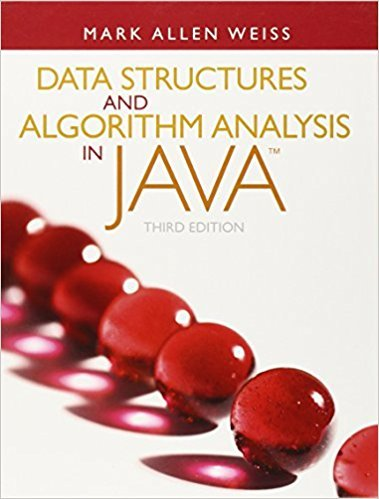×
Get Full Access to Data Structures And Algorithm Analysis In Java - 3 Edition - Chapter 9 - Problem 9.5
Get Full Access to Data Structures And Algorithm Analysis In Java - 3 Edition - Chapter 9 - Problem 9.5

×

# a. Find the shortest path from A to all other vertices for the graph in Figure 9.82.bISBN: 9780132576277 316

## Solution for problem 9.5 Chapter 9

Data Structures and Algorithm Analysis in Java | 3rd Edition

• Textbook Solutions
• 2901 Step-by-step solutions solved by professors and subject experts
• Get 24/7 help from StudySoup virtual teaching assistantsData Structures and Algorithm Analysis in Java | 3rd Edition

4 5 1 422 Reviews
26
3
Problem 9.5

a. Find the shortest path from A to all other vertices for the graph in Figure 9.82.b. Find the shortest unweighted path from B to all other vertices for the graph inFigure 9.82.

Step-by-Step Solution:
Step 1 of 3

NAME CONVERGENCE TESTS FOR INFINITE SERIES COMMENTS STATEMENT Geometric series ! ar k = 1 , if –1 < r < 1 Geometric series converges if and diverges otherwise –1 < r < 1 Divergence test – r If p If lim a k= 0, !a kay or may not converge. (nth Term test) lim ak " 0, then !a kiverges.  ! k  ! Integr If p is a real constant, the series ! 1 = + + . . . + + . . . – series a p p p p converges if p > 1 and diverges if 0 < p # 1. !a has positive terms, let f(x) b as tnhcitens aht ns fu(lis heeans yk tios integrate. This k e p\$l a beyn in the formula for u . kIf is decreasing and continuous for !a 1% Comparison test (Direct)  test only applies to series with positive terms. bok acdn vfe(g)e doxr bo th diverge. If !a k and !b k are series itshehsisivset ta s ssute hratt ache trm stnr !eak ften i le) sihae "itisg cgesrpes"d i!nbg term in !bk, then series" !a k converges, then the "smaller easier to apply. This test only applies to series Limit Comparison test (b) if the "smkller verieess". !a with positive terms. series" !b k diverges, then the "bigger diverges. k If !a k and !b kre series with positive terms su c h Tthhaits is easier to apply than the comparison test, if L > 0, =e n then both series converge or both diverge. k  ! b k but still requires some skill in choosing the Ratio test if L = 0, and series !b kor comparison. if L = +% a!nbdk! bconverges, then !a konverges. k diverges, then !a dkverges. If !a k is a series with positive term s srch t hti st when a lim ak+1 powers. k involves factorials or k th Root test k he! ifaL < 1 t ,e series converges k if L > 1 or L = +%, the series diverges if L = 1, another test must be used. If !a k is a series with positive term s ucry hist test when a if L < 1a te s eries (a )erge s= L, then  ! k k  ! k Alternating Series test k involves k th powers. if L > 1 or L = +%, the series diverges if L = 1, another test must be used. The series a Alternating Series Estimation Theor eIm f t:he alternating series ! ( conv er agif a – a + . . . and –a + a – a + a – . . . k+1 1 2 3 4 1 2 3 4 converges, then the truncat– io1n) errk ar for the n partial sum is less than a th (1) a if an alternating series cn+1 , e e.s, then the error (Leibniz's Theorem) The 1eri e2s d3i vaer > . . . and (2) ak = 0  ! in estimating the sum using Absolute Convergence and terms is less than the n+1 term. ges if lim ak " 0 k  ! If !a ik ! |ias a series with nzeortoe tt sih asrioe ceogese,tes na:bsolutely, then it if |aerges, i.e. | converges, then !a converges. if !ka | converges, then !a kconverges absolutely. k k Conditional Convergence Otherwikse,i arges, then !a konverges conditionally. diverges. k

Step 2 of 3

Step 3 of 3

##### ISBN: 9780132576277

The full step-by-step solution to problem: 9.5 from chapter: 9 was answered by , our top Science solution expert on 03/02/18, 06:05PM. Data Structures and Algorithm Analysis in Java was written by and is associated to the ISBN: 9780132576277. This textbook survival guide was created for the textbook: Data Structures and Algorithm Analysis in Java, edition: 3. This full solution covers the following key subjects: . This expansive textbook survival guide covers 12 chapters, and 457 solutions. Since the solution to 9.5 from 9 chapter was answered, more than 454 students have viewed the full step-by-step answer. The answer to “a. Find the shortest path from A to all other vertices for the graph in Figure 9.82.b. Find the shortest unweighted path from B to all other vertices for the graph inFigure 9.82.” is broken down into a number of easy to follow steps, and 33 words.

## Discover and learn what students are asking

Statistics: Informed Decisions Using Data : Organizing Qualitative Data
?In a relative frequency distribution, what should the relative frequencies add up to?

Unlock Textbook Solution